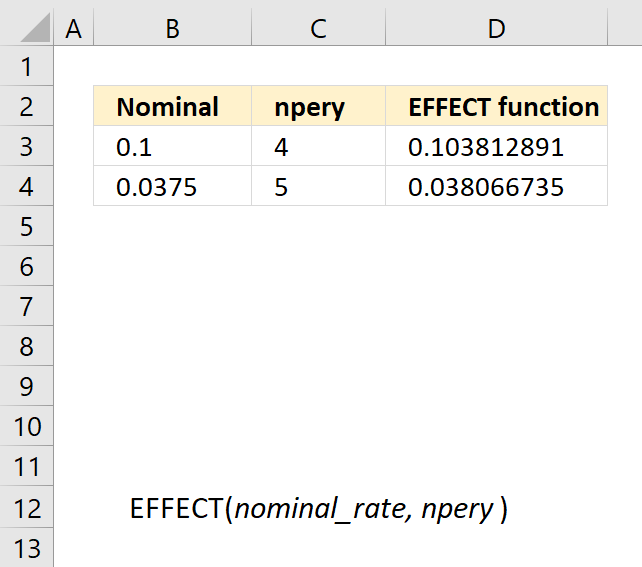Author: Oscar Cronquist Article last updated on May 11, 2022The EFFECT function calculates the effective annual interest rate, given the nominal annual interest rate and the number of compounding periods per year.

Formula in cell D3:

=EFFECT(B3,C3)

### Excel Function Syntax

EFFECT(nominal_rate, npery)

### Arguments

 nominal_rate Required. The nominal interest rate. npery Required. The number of compounding periods per year.